Markets
Futures
Earn
More
Cancel
FAQAccount FunctionsTutorialBinance Fan TokenBinance EarnCrypto Deposit/Withdrawal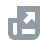Crypto Derivatives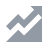FinanceAPISecurityOther Topics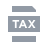Tax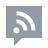Feed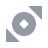Binance Convert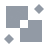NFTVIP
Home
Support Center
FAQ
Crypto Derivatives
Futures Contracts
USDⓈ-M Futures Contracts
Mark Price in USDⓈ-Margined Quarterly Futures

# Mark Price in USDⓈ-Margined Quarterly Futures

2021-09-09 08:23
Traditionally, the price of a quarterly futures contract will converge with its corresponding spot price as the contract expires after the three-month period. As the contract runs down towards expiry, the Mark price will closely reflect spot prices, and the moving average basis component will no longer be part of the Mark price calculation. This means that the Mark price of a quarterly futures contract will be computed differently as it reaches the time of expiration.

### .css-1lohbqv{box-sizing:border-box;margin:0;min-width:0;font-weight:600;}Before delivery date:

Mark Price = Price Index + Moving Average (5-minute Basis)*
Moving Average:
*The Moving Average (5-minute Basis) is calculated by taking the average of the bid and ask prices, subtracting the Price Index, and then taking the average of that value over the last 5 minutes. This calculation is done every 5 seconds, resulting in 60 data points.
Moving Average (5-minute Basis) = sum of [(Bid1_i + Ask1_i) / 2 - PI_i] / 60

### On the delivery date:

i) The time to delivery is greater than 1 hour
Using BTCUSDT 0924 as an example:
Mark Price before 24 September 2020, 06:59:59 UTC
= Price Index + Moving Average (5-minute Basis)*
*Moving Average (5-minute Basis) = Moving Average ((Bid1+Ask1)/2- Price Index), measures every minute in a 5-minute interval
During the system upgrade or the system down that pending all trading activities, the Mark price of a Quarterly Futures Contract whose time to delivery is greater than 1 hour is calculated as follows:
Keep the Mark price formula but use the Bid1 and Ask1 at the system stop time to calculate Moving Average (5-minute Basis) until the system is back to normal.

## How to Calculate Mark price (time to delivery is greater than 1 hour)

### Step 1: Calculate the Price Index

Assume Binance uses an equally-weighted price average; the prices of BTCUSDT trading pairs on the selected exchanges are 10,000 USDT, 10,0001 USDT, 10,0002 USDT, 10,003 USDT, and 10,004 USDT, respectively.
Price Index = (10,000 + 10,001 + 10,002 + 10,003 + 10,004) / 5 = 10,002 USDT

### Step 2: Calculate the Moving Average on 5-minute Basis

The Moving Average (5-minute Basis) is calculated by taking the average of the bid and ask prices, subtracting the Price Index, and then taking the average of that value over the last 5 minutes. This calculation is done every 5 seconds, resulting in 60 data points.
Moving Average (5-minute Basis) = sum of [(Bid1_i + Ask1_i)/2 - PI_i] /60
To calculate the moving average, we need to get the mid-price from the order book and Price Index of the first second of every 5 seconds for the past 5 minutes, then we will have n=60 in total.
For example, if we want to calculate the Mark Price of BTCUSDT 0924 at 12:30:00 UTC, the Mid-Price and Price Index are as follows:
Moving Average (5-minute Basis)
= Moving Average (Mid-Price - Price Index)
= [(Mid-Price - Price Index)1 + (Mid-Price - Price Index)2 + … + (Mid-Price - Price Index)60] / 60
= [(10,003 - 10,001) + (10,004 - 10,002) + … + (10,005 - 10,006)] / 60

### Step 3: Substitute the Price Index and Moving Average (5-minute Basis) into the formula

Let say Price Index = 10,002 USDT and Moving Average (5-minute Basis) = -1
Mark Price at 12:05:00 UTC
= Price Index + Moving Average (5-minute Basis)
= 10,002 USDT - 1 USDT
= 10,001 USDT
ii) Time to delivery is equal or less than 1 hour
Mark Price on 24 September 2020, 07:00:00 - 07:59:59 UTC
= Average of Price Index (every second from 07:00:00 and 07:59:59 UTC on the delivery day)

## How to Calculate Mark price (time to delivery is equal or less than 1 hour)

### Step 1: Calculate the Price Index

Assume Binance uses an equally-weighted price average; the prices of BTCUSDT trading pairs on the selected exchanges are 10,000 USDT, 10,0001 USDT, 10,0002 USDT, 10,003 USDT, and 10,004 USDT, respectively.
Price Index = (10,000 + 10,001 + 10,002 + 10,003 + 10,004) / 5 = 10,002 USDT

### Step 2: Calculate the Average of Price Index

Mark Price at Time n
= (Price Index 1 + Price Index 2 + … + Price Index n) / n
Example:
Mark Price at 07:00:02 on 24 September
= (Price Index at 07:00:00 + Price Index at 07:00:01 + Price Index at 07:00:02) / 3
= (10,002 + 10,003 + 10,004) / 3
= 10,003